"All the World's a Stage We Pass Through" R. Ayana

## Monday, 19 July 2010

### The Wave Structure of Matter

The Wave Structure of Matter

Wave Diagrams
by Geoff Haselhurst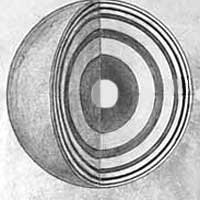I have spent a bit of time over the past 5 years slowly trying to improve these wave diagrams (it is very hard to draw spherical waves on a flat computer screen!). I think this page is now actually very useful for visualizing the Wave Structure of Matter (WSM) and how matter exists and moves about in Space. It also provides simple sensible solutions to a lot of the problems of physics caused by the discrete 'particle' conception of matter in space-time.
The comments and diagrams below relate to the most simple form of matter, the electron or positron (antimatter is just the opposite phase standing wave to matter - which is why they annihilate due to destructive interference).
The proton and neutron are more complex wave structures that need further study (I think they are formed from a collection of positrons and electrons which can form either a neutral positronium or the charm quark - but a proton has one more positron than electron obviously to explain positive charge).Please also realise that we are representing spherical waves in Space (imagine a soap bubble). These diagrams are 2 dimensional circular cross sections of the spherical wave (a limitation of working on a computer screen obviously).
I hope this page helps you better visualise the wave structure of matter. It takes time for our minds to adjust to new knowledge, so you need to think about it for a while! But it is simple and obvious once known.
Finally, I have just finished writing the following three pages - they are very useful for understanding the wave structure of matter, why I am convinced it is correct.
Truth Statements on Physical Reality - We can understand physical reality - matter is made of waves in space. These truth statements show people how to deduce physical reality for themselves and confirm it is true. It also provides a very concise summary of the central things the WSM explains. See if you can show any statement is not true.
Wave Equation in an Elastic Wave Medium - Deducing the famous energy equations, Einstein's E=mc2, Planck's E=hf and Newton's F=ma from simple wave equations in an elastic medium (space). This confirms that the equivalence of matter and energy is because matter is made of waves, and waves propagate energy. All forces are due to changes in wave velocity.
The Laws of Nature - The Wave Structure of Matter (WSM) deduces the laws of Nature that have been empirically observed and quantified over the past several centuries. This changes the foundations of science / physics from inductive (uncertain) to deductive (certain).
Any thoughts, comments, help with wave diagrams appreciated.
Geoff Haselhurst - Email

### Spherical Standing Wave (Two Dimensional Cross Section)

The wavelength of an electron is about 10-12 meters, i.e. there are about a thousand billion waves per meter, about a million million (trillion) wave center 'particles' in a pin head.
The frequency is about 1020 Hz (cycles / second) so they are vibrating very very quickly!! Given film shown in a movie theater is around 24 frames / second and appear as continuous motion, you then realise why we see matter as a continuous motion as well.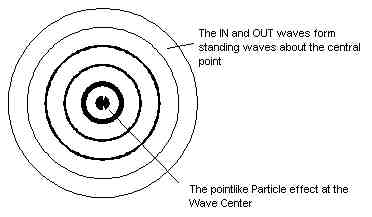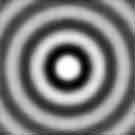Spherical Standing Wave

This diagram shows how the In-Waves and Out-Waves form a Standing Wave around the Wave-Center 'particle' [see flash movies at original link at end of article - Ed].
The wave velocity is the velocity of light c (300 million meters / sec) which equates to 1 second for your spherical in-waves to come in from the distance of the moon, about 8 minutes from the sun, 4 years from the nearest star, 100,000 years from across our milky Way galaxy, and about 15 billion years from across the observable universe.
Though we always measure this velocity to be the same (Einstein's special relativity) it does actually change - but so does the wavelength and thus the dimension which causes it to be measured the same (Nature is deceptive!)!
The wave velocity depends on wave amplitude (higher = faster) - this explains charge.
The wave velocity also slows slightly with higher mass density (more matter waves in space, calculated by squaring the wave amplitude). This is the cause of gravity and why light curves past the sun. (See light, charge and gravity diagrams below.)

### Spherical Standing Wave (Three Dimensional / Spherical Effect)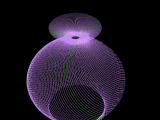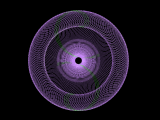These images show some interesting ways to get a spherical effects (though not really relevant to WSM)...

### Formation of Standing Waves - Representing Waves as Transverse

Like water waves, waves on a string - bit misleading compared to real waves in space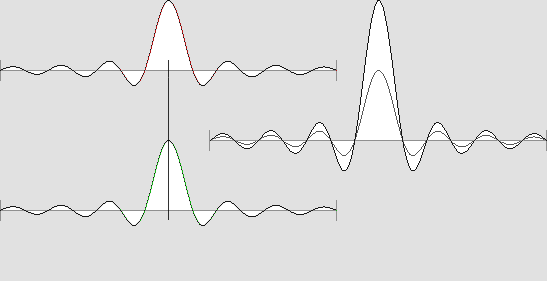### Cosmology: Source of Spherical In-Waves, Huygens Principle

 This shows how other matter's spherical Out-[See this at at the site link below] Waves form our matter's In-Waves (Huygens' Principle). Four important points!i) In reality there are about 1080 other wave center 'particles' whose spherical out-waves form into our spherical in-waves.ii) They are obviously not all the same distance away, but distributed throughout the space of our finite spherical observable Universe (Hubble Sphere) within infinite eternal Space.iii) These other wave-center 'particles' around us are also formed from the matter waves around them, and this process extends to infinity.iv) The diagram is misleading in showing the waves starting. In reality the waves are continually flowing out from other matter around us. i.e. The system is perpetual, the in and out waves are always being shared between electron wave-centers wherever you are in infinite space.So this is a very simplistic diagram of what is really going on. Electrons are very large complex wave structures of the universe (this is true for humans too!).
The spherical in-waves are formed from out waves of about 1080 other wave center 'particles' that exist in our observable universe within infinite space. Thus each wave center 'particle' is at the center of its observable universe within infinite space.
So matter (including all of us humans!) is really very large - spatially extended structures of the observable universe within infinite space. But we only 'see' the high wave amplitude wave center 'particles' and have been deluded into thinking matter was very small (the whole problem of modern physics - as they could not explain how matter was connected to other matter across the observable universe).
Albert Einstein realised there were no particles, that matter space and time are a unity. He just made the mistake of working with continuous fields in space-time, rather than real waves in Space.
Below is a slightly different method of showing this same Huygens principle and how our spherical in waves are formed from other matter's out waves. Basically a spherical surface approximates a planar surface if the sphere is large (just think of the surface of the earth which seems pretty flat / planar to us).
So you can get the same spherical In-Wave effect by using plane waves coming in from all directions around the wave center 'particle' as the following diagrams show.

Below are some stationary images of the electron wave center 'particle'.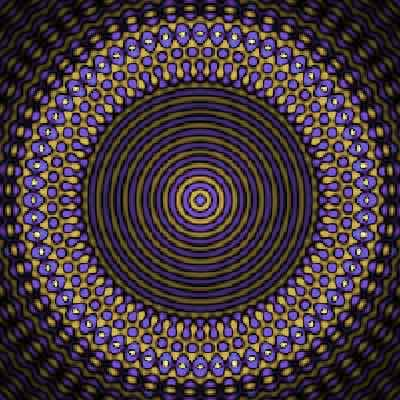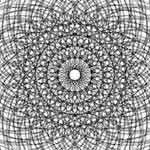### Cosmology: Cause of the Redshift with Distance

In WSM Cosmology, the observable universe is just a finite spherical region of infinite eternal space. Thus there is no need for an expanding universe, as other matter around our observable universe prevents it from collapsing. This is the equivalent of Einstein's Cosmological / Antigravity constant, but it is just normal gravity of matter outside our finite spherical observable universe within infinite space.
So why the redshift with distance?
Because as we look at matter further away from us, we find that we share less overlap of a common finite spherical observable universe. And this means that there is less energy exchange, which equates to a redshift with distance.

See The Cosmological Redshift Explained by the Intersection of Hubble Spheres
This article shows that each wave center 'particle' is the center of its finite spherical observable universe (Hubble Sphere). As two wave center 'particles' move apart there is less overlap of common Hubble spheres / observable universes, thus less wave interactions with increasing distance, thus less energy exchange which then explains the redshift with distance.

### Non Linear Wave Interactions, Formation of Coherent In-WavesThe above diagram represents a wave in a linear wave medium - thus the waves maintain their original velocity even as they flow through one another.
However, Space must be a non-linear Wave Medium to explain wave interactions. I think this means that when the above two waves flow through one another, they get stuck together as one wave with a combined wave amplitude and a higher velocity (i.e. Property of Space that waves with higher wave amplitude have higher velocity.) This is very important, as it is the source of all wave interactions, the cause of all forces, and explains how our In-Waves can form in a coherent way from other matter's out waves in the universe around us.

### The Motion of the Wave-Center 'Particle'

For a moving wave center 'particle' the spherical in-wave forms in one position in space (as waves combine), then disappears (as waves cancel) then the next spherical in-wave forms in a slightly different position, and so on ....
So matter really moves in discrete little steps (like frames of a film) but it appears continuous due to the high 'frame rate' / frequency. This is what quantum theory discovered - but never understood! The gravity image below shows this effect.

### Gravity

Another very approximate wave diagram which only shows the In-Waves (does not show Out-Waves) and the ellipsoidal shape is not accurate. But the basic idea of gravity being caused by slower wave velocity in higher energy / mass-energy density space is important.
This explains why Albert Einstein's general relativity is founded on maths describing the curvature of a sphere (Riemann, Gauss).

### Light

Light is not a discrete 'particle' - which is a theoretical interpretation of the empirical fact that light energy is discrete.
Light is really caused by the resonant coupling of two bound wave-centers of Spherical Standing Waves (Electrons) with oscillating wave functions. Because standing wave patterns only form at discrete frequencies the energy states of electron in atoms are discrete, and can only change by discrete amounts. This is why wave equations were first introduced into quantum theory (de Broglie, Schrodinger) as these were the only natural phenomena that behaved discretely.

### The de Broglie Wave is a High Velocity Phase Wave

The de Broglie wavelength is important as it is one of the fundamentals of Quantum theory that the WSM deduces due to Doppler shifts of two wave centers in relative motion. Interestingly the spherical wave interactions actually create a planar de Broglie wave (you can almost see this effect in above rough diagram).
Very importantly for EPR / non-locality, these beat waves travel at very high velocity for low relative motion v. i.e. phase wave velocity = c2 / v.
This is even more important when you realise that in these same wave equations for relative motion you also get the relativistic mass increase of Einstein's special relativity. This is remarkable - to unite central elements of the two main theories of modern physics from one set of wave equations (see work of Milo Wolff).

### Formation of Solid Bodies from Waves in Nearly Rigid Space

This is a flash movie i made a couple of years ago. It is pretty amateur but helps explain how waves can form solid bodies.
Space itself is very very rigid (to explain such high velocity waves). It does not 'flow' like water - it is a continuously connected wave medium (there are no parts / particles in its structure). Matter is really just spherically vibrating Space. This is why all matter vibrates and has a resonant frequency.

by Geoff Haselhurst

For further enlightenment see –

The Her(m)etic Hermit - http://hermetic.blog.com